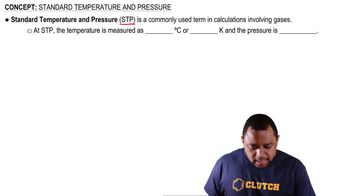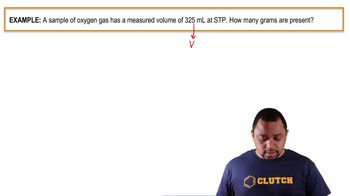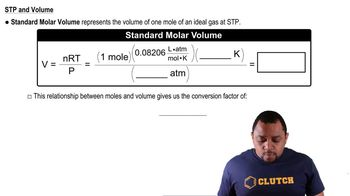Start typing, then use the up and down arrows to select an option from the list.
3:05 minutes
Problem 117
Textbook Question

# The Earth’s atmosphere has a mass of approximately 5.15 * 1015 kg. If the average molar mass of air is 28.8 g/mol, how many moles of gas make up the atmosphere? What is the volume of the atmosphere in liters under conditions of STP? (Note: The average molar mass of air is the weighted average of the molar mass of nitrogen and oxygen. 0.20132.0 g>mol2 + 0.80128.0 g>mol2 = 28.8 g>mol.)Verified Solution
This video solution was recommended by our tutors as helpful for the problem above.
150views

### Watch next

Master Standard Temperature and Pressure with a bite sized video explanation from Jules Bruno

Start learning00:3302:2001:2101:44